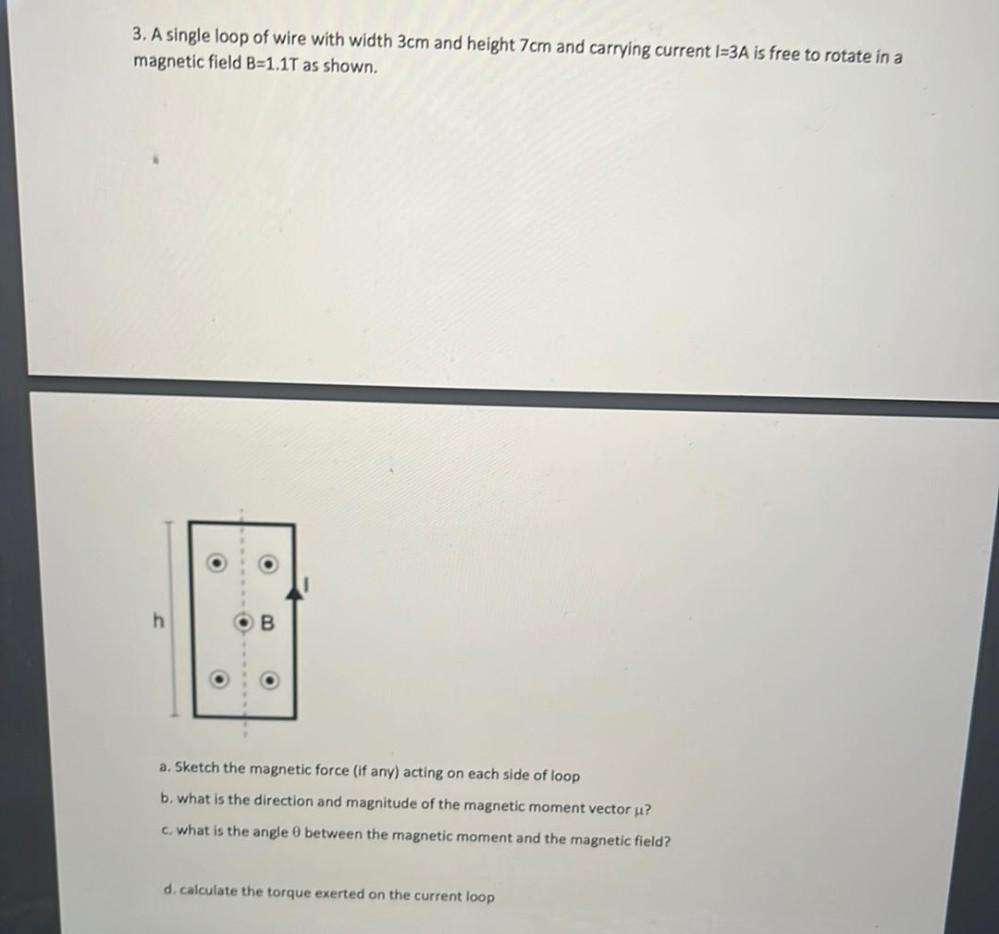Question:

# 3. A single loop of wire with width 3cm and height 7cm and carrying current 1=3A is free to rotate in a magnetic field B=1.17 as3. A single loop of wire with width 3cm and height 7cm and carrying current 1=3A is free to rotate in a magnetic field B=1.17 as shown. O h . o o o a. Sketch the magnetic force (if any) acting on each side of loop b. what is the direction and magnitude of the magnetic moment vector u? c. what is the angle between the magnetic moment and the magnetic field? d. calculate the torque exerted on the current loop